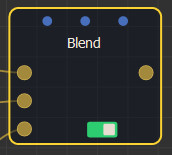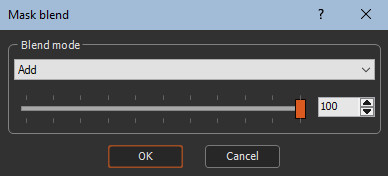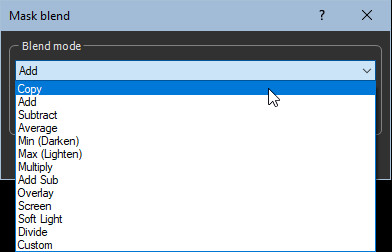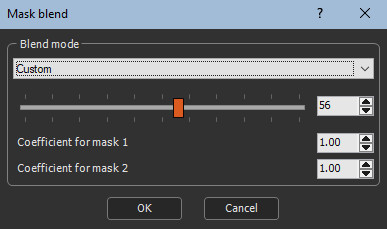This node creates a linear composition between two masks. For each value, the value of the output mask is equal to the value of the first input mask multiplied by the first coefficient, plus the value of the second input mask multiplied by the second coefficient. The second input mask is scaled to fit the size of the first input mask. The third input mask corresponds to the coefficient of the manual blend.

To add a node, right-click in the Graph Editor and select Create Node  > Mask Composition > Blend or use the keyboard shortcut NMBL (Node Mask BLend).Double click on the node to open its parameters:### Editing a mask Blend node

The blend option which indicates how the second mask is blended with the first mask using blending coefficient from 0 to 100.

• Â When the blending coefficient is 100, blending is carried out in its entirety.
• Â When the blending coefficient is 0, no blending is carried out and the result is the same as the first input mask.

When an optional third mask is connected, the value of this mask at each of its points is multiplied by the selected blending coefficient to obtain the final blending coefficient. For example, if a blending coefficient of 80 is selected and the optional mask contains values equal to 0.5, the final blending coefficient of 0.5 x 80 = 40 is achieved.

• An entirely black mask results in no blending, which is the same result as the first input mask.
• Similarly, an entirely white mask has no influence on the blending coefficient and the same result is obtained as if no mask is connected.

To edit the node, choose one of the following options from the drop-down list:• Copy: The second mask is copied over the first mask. This option is useful when a blending coefficient other than 100 is selected, or when an optional mask is connected. For example, with a blending coefficient of 25, 1/4 of the first mask and 3/4 of the second mask is obtained. With a blending coefficient of 50, a balanced blend of the two masks is achieved.
• Add: The output mask is the sum of the two input masks. If the sum is greater than 1, the value 1 (white) is selected. This is the default option.
• Subtract: The output mask is the difference between the first mask and the second mask. If the difference is less than 0, the value 0 (black) is selected. T

The same result is obtained as using the Copy option with a blending coefficient of 50.

• Min (Lighten): At each point, the output mask is equal to the lowest input mask.
• Max Darken): At each point, the output mask is equal to the highest input mask.
• Multiply: At each point, the value of the output mask is equal to the product of the values of the two masks.
• Add Sub:Â Â If the value of the second mask is greater than or equal to 0.5, a result equivalent to that of the Add option is obtained.Â If the value of the second mask is less than 0.5, a result equivalent to that of the Subtract option is obtained.
• Overlay: Combines the Multiply and Screen options. If the value of the second mask is greater than or equal to 0.5, a result equivalent to that of Screen option is obtained.Â If the value of the second mask is less than 0.5, a result equivalent to that of the Multiply option is obtained.
• Screen: The values of both input masks are inverted (black becomes white and vice versa) and are multiplied. The result are inversed to obtain the final value.Â Value of the output mask = 1 - (1 - Value of the input mask 1) x (1 - Value of the input mask 2)
• Soft Light: Creates a subtle lighter or darker result depending on the brightness of the first mask. If the value of the second mask is greater than or equal to 0.5, a result equivalent to that of the Max option is obtained.Â If the value of the second mask is less than 0.5, a result equivalent to that of the Min option is obtained.
• Divide: At each point, the output mask is equal to the value of the first mask divided by the value of the second mask. Â If the result is greater than 1, the value 1 (white) is selected. If the value of the second mask is 0 (black), the result is 1 (white).
• Custom:Â When this option is selected, two additional parameters appear:At each point, the value of the output mask is equal to the value of the first mask multiplied by the Coefficient for mask 1 parameter, plus the value of the second mask multiplied by the Coefficient for mask 2 parameter.Â Â If the result is less than 0, the value 0 (black) is selected.Â If the result is greater than 1, the value 1 (white) is selected.

Parameter Use